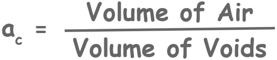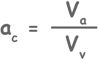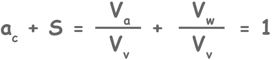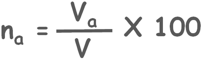Air Content And Percentage Air VoidsA soil mass in general is composed of three kinds of matter,

1. Solids

2. Liquid

3. GaseousSolids can be minerals or organic matters or both. These solids can be of different sizes and shapes and because of it they enclose empty spaces. These empty spaces are called pores or voids.

The proportions of solids, water and air in a soil mass influence its physical properties. And before founding any structure on the soil we should study the soil in detail including its physical properties.

To determine these physical properties of soil we need to learn few simple terms which are frequently used in soil engineering.

Lets discuss some of these soil properties:

Air Content

Air Content can be understood as the amount of soil pores present in the soil sample which are NOT filled with water or in turn are filled with air.

But different soils have different amount of voids and in turn different amount of air and water in it. So there is a need to define a term “Air Content” to know how much air is present in the voids of a sample.

Air content is denoted by ac and it is defined similar to the Degree of saturation.

Air content for a soil sample is defined as volume of air to the volume of voids that soil sample contains.Using 3 phase diagram of a partially saturated soil we can write air content of soil sample asSince we know that the definitions of air content and Degree of saturation are similar, we can see that Degree of saturation is defined for amount of water present in the soil while Air content is for amount of air present.

Unless the soil is in super saturated state air and water collectively makes the whole void space available, so summation of air content and degree of saturation will be equal to the unity.This relationship can be used to solve some mathematical problems.

Percentage Air voids

Percentage Air voids is denoted by na and is defined as the ratio of the volume of voids filled by air expressed as a percentage of the total volume of the soil mass.Do not get confused with the definitions of Air Content and Percentage Air voids.

Tags : air content, air void

Published on :2019-08-04